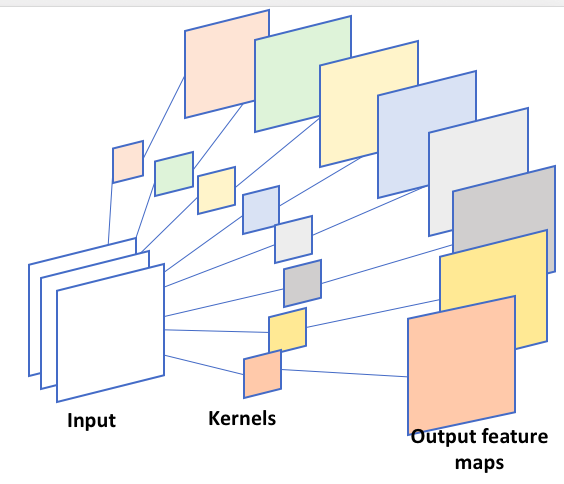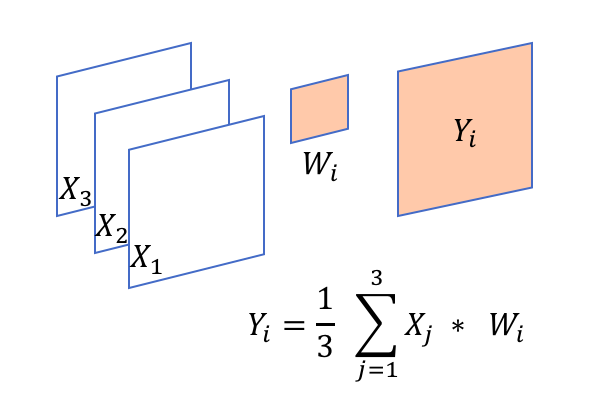# Conv2d certain values for groups and out_channels don't work

I am playing with the groups option in `torch.nn.Conv2d(..)`. It appears that both in_channels and out_channels must be divisible by groups. But in theory, it is not necessary, for example, if I have `in_channels=3`, and `groups=3`, then `out_channels=8` should give me the operation shown in the figure, but this will raise an error, saying “out_cnhannels is not divisable by groups”.

This works for in_channels=3, out_channels=9, and groups=3:

``````>>> conv = torch.nn.Conv2d(in_channels=3, out_channels=9,
kernel_size=(3,3), stride=1,
groups=3, bias=True)

>>> print(conv.weight.data.size())
torch.Size([9, 1, 3, 3])
``````

So, I think `out_channels=8` should work as well. Isn’t that right?1 Like

From what I understand, the number of channels in one kernel tensor is equal to the number of channels in the input image. E.g., if you set

``````c = torch.nn.Conv2d(in_channels=4, out_channels=8, kernel_size=(3, 3), groups=1)
``````

you will have 8 kernel tensors with 4 channels each, corresponding to the following scenario:

Then, if you change it to

``````c = torch.nn.Conv2d(in_channels=4, out_channels=8, kernel_size=(3, 3), groups=2)
``````

the 8 kernel tensors will be divided such that 2 of them are used for the first 2 image channels, and 2 of them are used for the other 2 image channels. The results are stacked, so if each kernel tensor has 4 channels, you have 2*4=8 output channels and 2 of them will be used for the other 2 image channels.

Now, if you change it to sth like this

``````c = torch.nn.Conv2d(3, 9, (3, 3), groups=3)
``````

you would end up with 1 kernel channel per image channel:

Finally, if you write sth like you suggested:

``````c = torch.nn.Conv2d(in_channels=3, out_channels=8, kernel_size=(3, 3), groups=3)
``````

the code will complain, because it wouldn’t now how to evenly distribute the 8 kernels among the 3 input image channels. Eg., the first channel might get 3 kernels, the 2nd gets 3, and the third gets 2? That’s possible but ambiguous.

7 Likes

@smth
Hm, I was just thinking that it would maybe help to have a parameter called `num_kernel_channels_per_input_channels` (or probably a shorter name ;)) instead of `groups` in future? The default could be

``````num_kernel_channels_per_input_channels = None
``````

which would set `num_kernel_channels_per_input_channels` equal to the number of channels in the input image. This could make the scenarios above a bit more intuitive and probably more user-friendly.

I see; I thought that each kernel would be shared with all three input channels so given kernel W_k and the 3 input channels X1 X2 and X3, we could have the following:Hmm, I don’t think that’s possible in PyTorch without workarounds, but maybe someone else knows more …

Eg as a work around, you could split the input image into 3 matrices, and for each matrix, you could use the same convolutional layer. And then, you could average the results over the three outputs. In pseudo code, sth like this:

``````x1 = X[:, 0, :, :]
x2 = X[:, 1, :, :]
x3 = X[:, 2, :, :]

o1 = conv2d_1(x1, in_channels=1, out_channels=1...)
o2 = conv2d_1(x2, in_channels=1, out_channels=1...)
o3 = conv2d_1(x3, in_channels=1, out_channels=1...)

o = (o1 + o2 + o3) / 3
``````

you can do that by folding the channels dimension into batch dimension, and unfolding after finishing.
For example:

``````input = Tensor(N, C, H, W)
layer = nn.Conv2d(1, 1, kernel_size)

# first fold C into N
input = input.view(N*C, 1, input.size(2), input.size(3))
out = layer(input)

# unfold
out = out.view(N, C, out.size(2), out.size(3))
``````
4 Likes

Current docs don’t mention that out_channels should also be divisible by the number of groups:

https://pytorch.org/docs/0.1.12/_modules/torch/nn/functional.html#conv2d